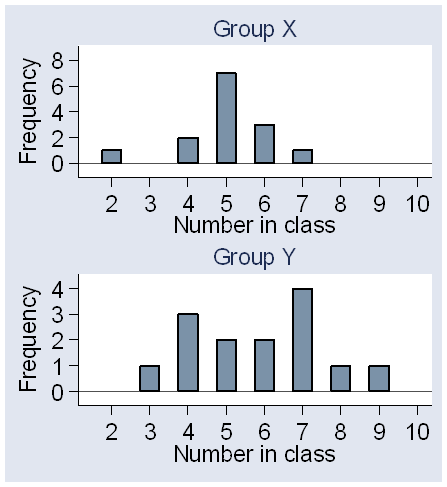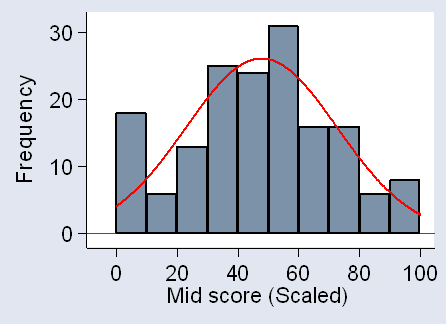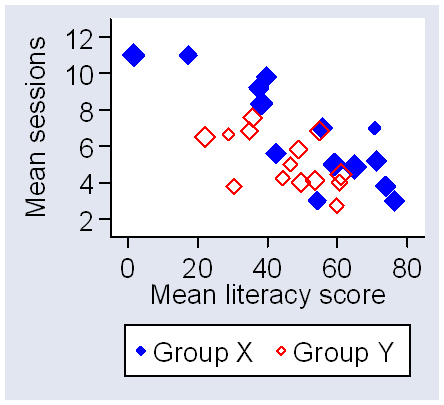# Analysis of a cluster-randomised trial in education

This is an expanded version of a talk given to the Workshop on Cluster Randomised Trials at the First Conference on Randomised Controlled Trials in the Social Sciences, University of York, September 2006.

## Introduction

This is a comparison of several analyses of a culster randomised trial in education. The data come from a trial of incentives to attend adult literacy classes, carried out by Greg Brooks, Carole Torgerson, Jeremy Miles, and David Torgerson.

There are two groups of 14 classes. The groups are labeled X and Y in this data set, which was blinded for analysis.
Group X: 77 students
Group Y: 86 students

The number of learners varied considerably between classes:The outcome variable is the number of sessions attended following randomisation. This has a distribution which has a spike at zero, where there were several students who did not attend again after randomisation, and otherwise is slightly skew to the right:For this analysis, we shall treat this as suitable for Normal theory analyses.

In this trial, literacy classes were randomised to incentive or no incentive. This was done after the class had begun, so there are no true baseline data, but we do have a literacy score at randomisaton, which may also be a predictor of sessions attended.The analysis below is presented in Stata, but could be done using a variety of software.

## The sample data

The key variables used in the analysis are available for download in several different formats:

Variables are:

1. group,
2. midscore (scaled),
3. sessions attended,
4. learner code,
5. class code.

Group is coded X=1, Y=2. Missing data are coded ".".

## Analysis ignoring clustering

First, we could compare the mean number of sessions ignoring the clustering, using the two-sample t method:

```. ttest sessions, by(group)

Two-sample t test with equal variances

------------------------------------------------------------------------------
Group |     Obs        Mean    Std. Err.   Std. Dev.   [95% Conf. Interval]
---------+--------------------------------------------------------------------
Group X |      70    6.685714    .4177941    3.495516    5.852238    7.519191
Group Y |      82    5.280488    .2991881    2.709263    4.685197    5.875778
---------+--------------------------------------------------------------------
combined |     152    5.927632    .2566817    3.164585     5.42048    6.434783
---------+--------------------------------------------------------------------
diff |            1.405226    .5037841                .4097968    2.400656
------------------------------------------------------------------------------
Degrees of freedom: 150

Ho: mean(Group X) - mean(Group Y) = diff = 0

Ha: diff < 0               Ha: diff != 0              Ha: diff > 0
t =   2.7893                t =   2.7893              t =   2.7893
P < t =   0.9970          P > |t| =   0.0060          P > t =   0.0030
```

This give us P = 0.006  a highly significant difference! But it is wrong  it ignores the clustering!

## Analysis using summary statistics

Whole classes were allocated to groups, so to be valid the analysis must take this into account. We could carry out an analysis using the class as the unit of analysis and the mean number of sessions for the pupils in the class as the outcome variable. This is the simplest valid anbalysis.

In Stata we create these means by the "collapse" command:

```
. collapse (mean) sessions group midscl (count) n=sessions, by(class)
```

This gives the following variables:
class, the code for the class,
sessions, the mean number of sessions for the class,
group, the code for the group,
midscl, the scaled literacy score at randomisation,
n, the number of learners in the class for whom we have data on sessions attended. (Note that the command "(count) n=sessions" gives n as a count of the number of learners for whom sessions is not a missing value.)

The analysis is:

```
. ttest sessions , by(group)

Two-sample t test with equal variances

------------------------------------------------------------------------------
Group |     Obs        Mean    Std. Err.   Std. Dev.   [95% Conf. Interval]
---------+--------------------------------------------------------------------
1 |      14     6.69932    .7457716    2.790422    5.088178    8.310461
2 |      14    5.189229    .3974616    1.487165    4.330565    6.047893
---------+--------------------------------------------------------------------
combined |      28    5.944274     .439363     2.32489    5.042776    6.845773
---------+--------------------------------------------------------------------
diff |            1.510091    .8450746                -.226985    3.247166
------------------------------------------------------------------------------
Degrees of freedom: 26

Ho: mean(1) - mean(2) = diff = 0

Ha: diff < 0               Ha: diff != 0              Ha: diff > 0
t =   1.7869                t =   1.7869              t =   1.7869
P < t =   0.9572          P > |t| =   0.0856          P > t =   0.0428
```

P = 0.0856  not significant. This is almost valid  it takes the data structure into account. However, the classes are of different sizes and this analysis gives them all equal weight. We should also take into account the variation in class size.

In Stata, we cannot weight the "ttest" command, but must use the regression command instead. If we do regression on the indicator variable for group, this gives exactly the results as the two sample t test:

```. regress sessions group

Source |       SS       df       MS              Number of obs =      28
-------------+------------------------------           F(  1,    26) =    3.19
Model |  15.9626158     1  15.9626158           Prob > F      =  0.0856
Residual |  129.975482    26    4.999057           R-squared     =  0.1094
-------------+------------------------------           Adj R-squared =  0.0751
Total |  145.938098    27  5.40511474           Root MSE      =  2.2359

------------------------------------------------------------------------------
sessions |      Coef.   Std. Err.      t    P>|t|     [95% Conf. Interval]
-------------+----------------------------------------------------------------
group |  -1.510091   .8450746    -1.79   0.086    -3.247166     .226985
_cons |    8.20941    1.33618     6.14   0.000     5.462853    10.95597
------------------------------------------------------------------------------
```

The group coefficient is the same as the difference between group means (except for the change in sign) and the P value is the same, P = 0.086.

We can compare the mean number of sessions per class by the regression method, weighted by class size:

```. regress session group  [aweight=n]
(sum of wgt is   1.5200e+02)

Source |       SS       df       MS              Number of obs =      28
-------------+------------------------------           F(  1,    26) =    2.90
Model |  13.7364762     1  13.7364762           Prob > F      =  0.1006
Residual |  123.192613    26  4.73817742           R-squared     =  0.1003
-------------+------------------------------           Adj R-squared =  0.0657
Total |  136.929089    27  5.07144775           Root MSE      =  2.1767

------------------------------------------------------------------------------
sessions |      Coef.   Std. Err.      t    P>|t|     [95% Conf. Interval]
-------------+----------------------------------------------------------------
group |  -1.405226   .8253046    -1.70   0.101    -3.101664    .2912116
_cons |   8.090941    1.33547     6.06   0.000     5.345843    10.83604
------------------------------------------------------------------------------
```

Now P = 0.101  not significant. The mean difference is also slightly changed, –1.41, i.e. the mean for Group X exceeds the mean for Group Y by 1.41 sessions. This analysis is valid  it takes the data structure into account, including the variation in class size.

## Analysis using robust standard errors

A different approach to the analysis is to use the individual student as the unit of analysis but to adjust the standard errors for the clustering. One way to do this is the robust standard error or Huber-White-sandwich method. We can do this using the regression command:

```. clear

. use incent2

. regress sessions group, robust cluster(class)

Regression with robust standard errors                 Number of obs =     152
F(  1,    27) =    2.79
Prob > F      =  0.1062
R-squared     =  0.0493
Number of clusters (class) = 28                        Root MSE      =  3.0958

------------------------------------------------------------------------------
|               Robust
sessions |      Coef.   Std. Err.      t    P>|t|     [95% Conf. Interval]
-------------+----------------------------------------------------------------
group |  -1.405226   .8407909    -1.67   0.106    -3.130387     .319934
_cons |   8.090941   1.535933     5.27   0.000     4.939466    11.24242
------------------------------------------------------------------------------
```

(In the Stata command, "robust" is unnecessary, as "cluster" implies it, but I have included it for clarity.)

For this analysis, P = 0.106  not significant, and the estimate is 1.41 in favour of Group X. The estimate is identical to the analysis using summary statistics weighted for class size, though the standard error, P value, and 95% confidence interval are very slightly different.

The analysis is valid  it takes the data structure into account.

Why should we do this rather than the summary statistics, if we get the same answer? Because it enables us to adjust for a covariate. This might explain some of the variation in attendance and hence improve the estimate of the difference between group means:

```regress sessions group midscl, cluster(class)

Regression with robust standard errors                 Number of obs =     152
F(  2,    27) =   11.91
Prob > F      =  0.0002
R-squared     =  0.1956
Number of clusters (class) = 28                        Root MSE      =  2.8572

------------------------------------------------------------------------------
|               Robust
sessions |      Coef.   Std. Err.      t    P>|t|     [95% Conf. Interval]
-------------+----------------------------------------------------------------
group |  -1.533053   .6128085    -2.50   0.019    -2.790433   -.2756742
midscl |   -.049151   .0104713    -4.69   0.000    -.0706363   -.0276658
_cons |   10.56678   1.304614     8.10   0.000     7.889936    13.24363
------------------------------------------------------------------------------
```

This analysis gives P = 0.019  significant.

This analysis is valid  it takes the data structure into account. It was the one done by Jeremy Miles for the final analysis of the trial. The adjustment produces a true significant difference.

## Adjustment when using summary statistics

We could do the same regression or analysis of covariance using summary statistics. We collapse the data, as before, to give for each class the mean number of sessions attended, the intervention group, the midcourse literacy score, and the number of learners for whom we have data:

```
. collapse (mean) sessions group midscl (count) n=sessions, by(class)

. regress session group midscl [aweight=n]
(sum of wgt is   1.5200e+02)

Source |       SS       df       MS              Number of obs =      28
-------------+------------------------------           F(  2,    25) =   22.97
Model |  88.6762362     2  44.3381181           Prob > F      =  0.0000
Residual |   48.252853    25  1.93011412           R-squared     =  0.6476
-------------+------------------------------           Adj R-squared =  0.6194
Total |  136.929089    27  5.07144775           Root MSE      =  1.3893

------------------------------------------------------------------------------
sessions |      Coef.   Std. Err.      t    P>|t|     [95% Conf. Interval]
-------------+----------------------------------------------------------------
group |  -1.778653   .5301429    -3.36   0.003    -2.870503   -.6868038
midscl |  -.0945941    .015181    -6.23   0.000    -.1258598   -.0633283
_cons |   13.13811   1.175841    11.17   0.000     10.71642     15.5598
------------------------------------------------------------------------------
```

Surprisingly, this works as well as, or even better than, the robust standard errors regression. We have a larger estimate of the difference with a smaller P value (0.003). The literacy score is a very strong predictor:(The area of the diamond is proportional to the number in the class.)

The summary statistics method often works very well.

## Finding the ICC and design effect

We can find the ICC easily in Stata using the "loneway" command (long one-way analysis of variance).

```. loneway sessions class

One-way Analysis of Variance for sessions: Sessions attended mid-post

Number of obs =       152
R-squared =    0.4916

Source                SS         df      MS            F     Prob > F
-------------------------------------------------------------------------
Between class          743.32934     27    27.530716      4.44     0.0000
Within class            768.8746    124    6.2006016
-------------------------------------------------------------------------
Total                  1512.2039    151    10.014596

Intraclass       Asy.
correlation      S.E.       [95% Conf. Interval]
------------------------------------------------
0.38856     0.09376       0.20480     0.57232

Estimated SD of class effect            1.985031
Estimated SD within class               2.490101
Est. reliability of a class mean         0.77478
(evaluated at n=5.41)
```

Hence ICC = 0.38856 = 0.39.

The design effect for constant cluster size is

1 + (m - 1)ICC,

where m is the cluster size. If we have varying cluster size, we replace m by

Sum mi2 / Sum mi = Mean mi + (k - 1) Var(mi) / (k Mean mi)

where k is the number of clusters. We can find the mean and variance of the mi from the summary data.

```. collapse (mean) sessions group (count) n=sessions, by(class)

. sum n

Variable |       Obs        Mean    Std. Dev.       Min        Max
-------------+--------------------------------------------------------
n |        28    5.428571    1.525792          2          9

. disp  5.428571 + (28-1)* 1.525792 ^2/( 28 * 5.428571)
5.8421047
```

Then we use ICC = 0.38856 to get the design effect:

```. disp 1 + (5.8421047 - 1)*0.38856
2.8814482
```

This design effect may be too large, because the difference between the groups is included in the difference between the classes. We can find an ICC for each group:

```. loneway sessions class if group==1

One-way Analysis of Variance for sessions: Sessions attended mid-post

Number of obs =        70
R-squared =    0.5984

Source                SS         df      MS            F     Prob > F
-------------------------------------------------------------------------
Between class          504.49524     13    38.807326      6.42     0.0000
Within class           338.59048     56    6.0462585
-------------------------------------------------------------------------
Total                  843.08571     69    12.218634

Intraclass       Asy.
correlation      S.E.       [95% Conf. Interval]
------------------------------------------------
0.52107     0.13054       0.26522     0.77692

Estimated SD of class effect            2.564807
Estimated SD within class               2.458914
Est. reliability of a class mean         0.84420
(evaluated at n=4.98)

.
. loneway sessions class if group==2

One-way Analysis of Variance for sessions: Sessions attended mid-post

Number of obs =        82
R-squared =    0.2763

Source                SS         df      MS            F     Prob > F
-------------------------------------------------------------------------
Between class          164.26465     13    12.635743      2.00     0.0341
Within class           430.28413     68    6.3277077
-------------------------------------------------------------------------
Total                  594.54878     81    7.3401084

Intraclass       Asy.
correlation      S.E.       [95% Conf. Interval]
------------------------------------------------
0.14624     0.10793       0.00000     0.35778

Estimated SD of class effect            1.041094
Estimated SD within class               2.515494
Est. reliability of a class mean         0.49922
(evaluated at n=5.82)
```

As a simple approximation, we can average these two ICCs: but the ICC is not much smaller. The design effect would be

```. disp 1 + (5.8421047 - 1)*.333655
2.6155924
```

giving 2.62 compared to 2.88.

## Analysis using the design effect

We can now multiply the standard error for our crude (invalid) t test by the square root of the design effect:

```. ttest sessions , by(group)

Two-sample t test with equal variances

------------------------------------------------------------------------------
Group |     Obs        Mean    Std. Err.   Std. Dev.   [95% Conf. Interval]
---------+--------------------------------------------------------------------
X |      70    6.685714    .4177941    3.495516    5.852238    7.519191
Y |      82    5.280488    .2991881    2.709263    4.685197    5.875778
---------+--------------------------------------------------------------------
combined |     152    5.927632    .2566817    3.164585     5.42048    6.434783
---------+--------------------------------------------------------------------
diff |            1.405226    .5037841                .4097968    2.400656
------------------------------------------------------------------------------
Degrees of freedom: 150

Ho: mean(X) - mean(Y) = diff = 0

Ha: diff < 0               Ha: diff != 0              Ha: diff > 0
t =   2.7893                t =   2.7893              t =   2.7893
P < t =   0.9970          P > |t| =   0.0060          P > t =   0.0030

. disp .5037841 * sqrt(2.8814482)
.8551649
```

and hence find a new confidence interval and P value. For the P value:

```. disp 1.405226/.8551649
1.6432223

. disp 2*ttail(152, 1.6432223)
.10240394
```

so the P value, P = 0.102, is very similar to the P = 0.106 by robust regression and P = 0.101 by summary statistics.

For the confidence interval:

```
. disp invttail(150,0.975)
-1.9759053

. disp 1.405226 - 1.9759053 * 0.8551649
-.28449886

. disp 1.405226 + 1.9759053 * 0.8551649
3.0949509
```

The 95% confidence interval is –0.28 to 3.09. Compare this to –0.32 to 3.13 by robust regression and –0.23 to 3.25 by summary statistics. They are very similar.

This cluster randomised trial has a very large cluster effect, as measured by the ICC = 0.39. This produces a substantial design effect, even though the clusters are fairly small. In turn, this leads to a misleading result if we ignore the clustering.

There are several ways in which we could do a valid analysis for these data and we have tried three of them: cluster summary statistics, robust standard errors, and the use of the design effect to adjust standard errors. These all gave very similar results. Even with the use of a covariate, we got similar results for robust regression and summary statistics.

In this case the covariate had a fairly strong predictive power for the outcome variable and also varied between clusters much more than the variation between individuals would lead us to expect (P<0.0001, one way anova of literacy score by class).

If anyone would like to try a multilevel or Bayesian hierarchical model on these data, I would be happy to add them to this comparison.

My conclusion for the moment is that, provided a method which takes the clustering into account is used, it does not matter which we use for the analysis of these data.

Back to clustered study designs menu.

Back to some papers and talks menu.